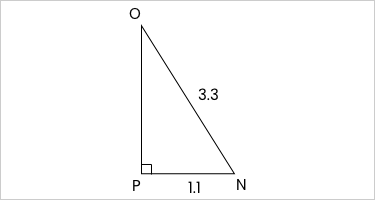### Still have math questions?

Q:

The maximum slope of a wheelchair ramp is 1:12. For a wheelchair ramp made with these specifications, what angles does the ramp make with the ground? Round your answer to the nearest hundredth.

Q:

In $$\triangle\text{NOP}$$, the measure of $$\angle\text{P}=90^{\circ}$$$$\text{PN}=1.1$$, and $$\text{NO}=3.3$$ feet. Find the measure of $$\angle\text{N}$$ to the nearest degree.Q:

This is an observation tower out in Blowing Rock, NC that is 40 feet tall. If you climb to the top and view someone's campfire at an angle of depression of 3 degrees, how far from the base of the tower is the campfire? Round your answer to the nearest foot.

Q:

A sun casts a shadow of a 50-foot building. If the angle of elevation to the sun is $$64^{\circ}$$, how long is the shadow? Round to the nearest tenth.

a. 24.4 feet

b. 114.0 feet

c. 102.5 feet

d. 55.6 feet

Q:

From the top of a building 21 m tall, the angle of depression to a car on the road below is 70 degrees. How far is the car from the base of the building? Sketch a diagram to help you...

a. 61 m

b. 57.7 m

c. 7.6 m

d. 19.7 m

Q:

When a coordinate grid is superimposed on a map of Lanesville, the middle school is located at (15, 23) and the library is located at (27,9). If each unit represents 1 mile,, how many miles apart are the middle school and the library? Round your answer to the nearest tenth.

Q:

In $$\triangle\text{MNO}$$, the measure of $$\angle\text{O}=90^{\circ}$$$$\text{ON}=35$$$$\text{NM}=37$$, and $$\text{MO}=12$$. What is the value of the tangent of $$\angle\text{M}$$ to the nearest hundredth?

Q:

In the triangle ABC, C=90, AC=3.2m and BC=4.8m. Find B.